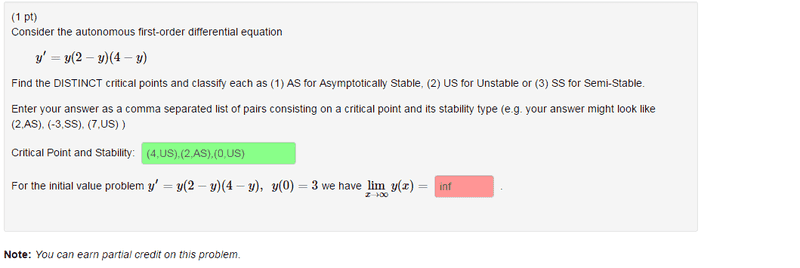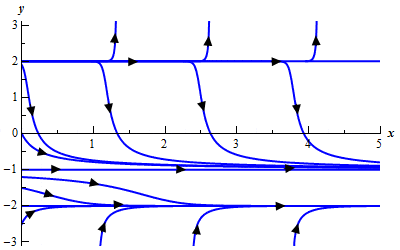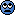# Find the limit of a given IVP

## Homework Statement

http://s14.postimg.org/an6f4t2ht/Untitled.png## The Attempt at a Solution

I'm not sure what they want me to do on the last part. I tried some googling and looking in my textbooks but I didn't find any examples.
It seems to me like the function goes to infinity if it's unstable as x -> inf.

Can somebody explain to me what exactly they want me to do?

HallsofIvy
Homework Helper
My suggestion would be that you actually solve the equation!
It is just $$\frac{dy}{y(y- 2)(y- 4)}= dx$$ so integrate both sides, using "Partial Fractions" on the left.

•Feodalherren
You have to consider the y value which the initial point passes through. In this case it passes through y = 3.

Once you figure this, see which asymptotes it lies between. Do you think it will approach the stable or unstable one?

The x-->Infinity is really just saying that a solution running through the point will go towards one of its asymptotes. It took me awhile to understand this back when I took DEs but the focus is on the y-value of the condition and y-value of asymptotes, not so much the x value

These implicit questions want you to understand the end behavior of the derivative without necessary knowing the explicit form of y(x)

•Feodalherren
I'm not sure I still really understand it, but at least I know what to do. Thanks!

I'm not sure I still really understand it, but at least I know what to do. Thanks!If you look at the picture, there are different solution curves based on the points which the curve passes through (IVP). As x goes to infinity, the curves will go toward or away an equilibrium point. In this case equilibrium points are y = 2, - 1, - 2. They are called equilibrium points because if the initial value y(x) = one of the equilibrium points, the solution will go toward that asymptote even if it is an unstable asymptote (these are straight lines going through each curve).

1. Which are stable, unstable, and semistable?
2. What will y approach as x - - >
infinity and y(0) = 2
3. What will y approach as x - - > infinity and y(4) = 0

Last edited:
epenguin
Homework Helper
Gold Member
My suggestion would be that you actually solve the equation!
It is just $$\frac{dy}{y(y- 2)(y- 4)}= dx$$ so integrate both sides, using "Partial Fractions" on the left.

IMHOAlways nice when you can, but isn't it more important and more often applicable and useful to be able to give these answers without solving the equation, because most times you can't?

I don't think the question/questioners were looking for the solution; the student could add it as afterthought to his answer to show off.